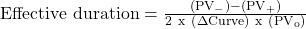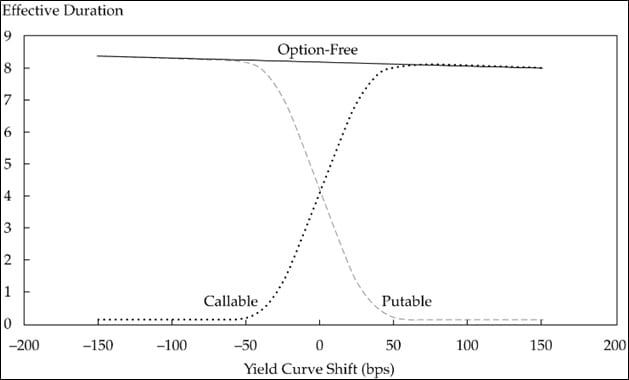Essential Concept 68: Duration | IFT World
101 Concepts for the Level I Exam

# Essential Concept 68: DurationEffective duration indicates the sensitivity of a bond’s price to a 100-bps parallel shift of the benchmark yield curve in particular, the government par curve; assuming no change in the bond’s credit spread.The flowing procedure is used to apply this formula in practice.

1. Given a price (PV0), calculate the implied OAS to the benchmark yield curve at appropriate interest rate volatility.
2. Shift the benchmark yield curve down, generate a new interest rate tree, and then revalue the bond using the OAS calculated in Step 1. This value is PV.
3. Shift the benchmark yield curve up by the same magnitude as in Step 2, generate a new interest rate tree, and then revalue the bond using the OAS calculated in Step 1. This value is PV+.
4. Calculate the bond’s effective duration.

The following figure compares the effective duration of option-free, callable and putable bonds.The effective durations of various types of instruments are shown in the table below.

 Type of Bond Effective Duration Cash 0 Zero-coupon bond ≈ Maturity Fixed-rate bond < Maturity Callable bond ≤ Duration of straight bond Putable bond ≤ Duration of straight bond Floater (Libor flat) ≈ Time (in years) to next reset